International
Tables for
Crystallography
Volume D
Physical properties of crystals
Edited by A. Authier

International Tables for Crystallography (2006). Vol. D, ch. 1.6, p. 176

## Section 1.6.8. Glossary

A. M. Glazera* and K. G. Coxb

aDepartment of Physics, University of Oxford, Parks Roads, Oxford OX1 3PU, England, and bDepartment of Earth Sciences, University of Oxford, Parks Roads, Oxford OX1 3PR, England
Correspondence e-mail:  glazer@physics.ox.ac.uk

### 1.6.8. Glossary

| top | pdf |,,refractive indices of biaxial indicatrix,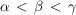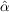polarizability operatorith component of magnetic induction c velocity of light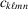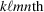component of elastic stiffness tensor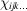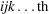component of generalized susceptibility d density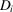ith component of dielectric displacementphase difference of light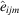unit antisymmetric pseudotensor of rank 3ith component of electric field,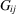ijth component of gyration tensor G gyration vector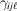third-rank optical gyration susceptibility H magnetic field intensityijth component of dielectric impermeability tensorpermittivity of free spaceijth component of dielectric tensorellipticity of wave k wavevector of light propagating in crystal (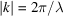)wavelength of light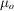vacuum magnetic permeability n refractive index of light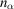,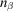,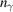refractive indices for biaxial indicatrix,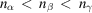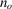ordinary refractive index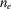extraordinary refractive index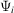wavefunction of state iith component of electric polarization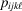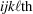component of elasto-optic (strain-optic) tensor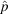electric dipole operatoroptical rotatory powercomponent of linear piezo-optic tensor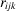ijkth component of linear electro-optic tensorunit vector in the direction of s, the wave normalijth component of strain tensorijth component of stress tensor v velocity of sound V half the angle between optic axescyclic frequencydirection of ith Cartesian axis, i = 1, 2, 3